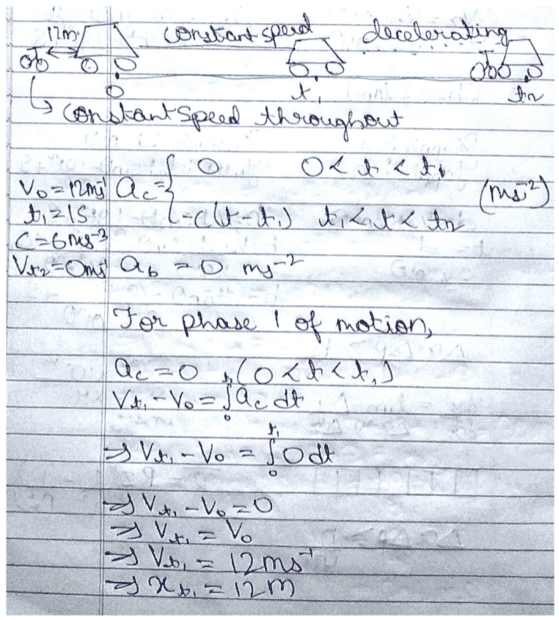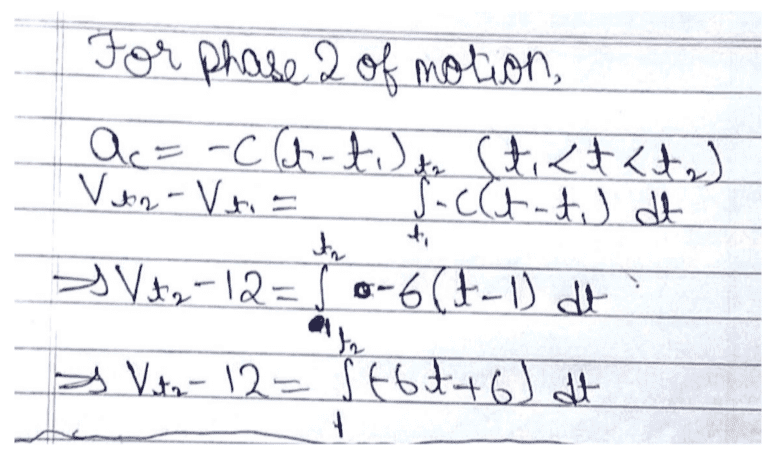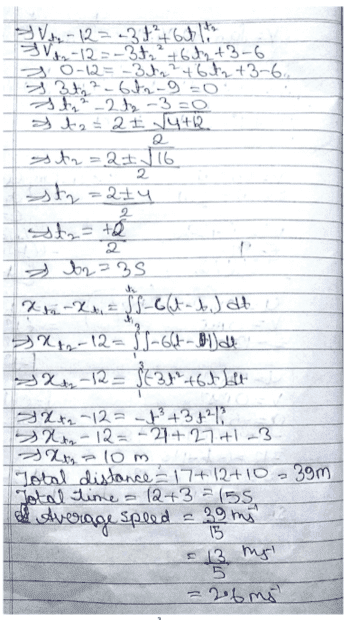# What is the speed of the bicycle?

• brochesspro
In summary, you seem to have pasted in an image containing your work rather than typing in your work directly. This is a common mistake and can make it difficult to understand what you are doing.
brochesspro said:

brochesspro said:
You need to post some Latex or, at least, an image of your work.

It has an image, if you can open it.

brochesspro said:
It has an image, if you can open it.

PeroK said:
Ok, but is that a rule?

brochesspro said:
Ok, but is that a rule?
Yes.Then what is the "Insert link" button used for?

brochesspro said:
There are a few mistakes there. ##t_2 = 3s## is correct. The calculation of ##x_{t_2}## has gone wrong somewhere. You added an extra ##12s## for some reason(?).

I added the 12 seconds by mistake. I should have added 1 second for the phase where the speed is constant.

PeroK said:
There are a few mistakes there. ##t_2 = 3s## is correct. The calculation of ##x_{t_2}## has gone wrong somewhere. You added an extra ##12s## for some reason(?).
I too feel I have gone wrong in that part.

brochesspro said:
I added the 12 seconds by mistake. I should have added 1 second for the phase where the speed is constant.
The way you did it, ##t_2## is the final time. You don't need to add anything.

brochesspro said:
I too feel I have gone wrong in that part.
I don't see the error immediately - but I used ##T## to keep things simple.

Oh, I see, so I did a mistake in something basic.

I think I neglected the constant ##C## while integrating for the first time for finding ##x_{t_2}##.

brochesspro said:
Oh, I see, so I did a mistake in something basic.
You have ##a(t) = -6(t - 1)## (let's do the maths without units). So: $$v(t) = -3t^2 + 6t + k$$ where ##k## is the constant of integration. We know that ##v(1) = 12## (note that we do not have ##v(0) = 12##). So:
$$k = 12 + 3 - 6 = 9$$ and $$v(t) = -3t^2 + 6t + 9$$ That gives ##v(3) = 0##. Integrating again:
$$x(t) = -t^3 +3t^2 +9t + l$$
I think that's where you went wrong, by losing the ##9t## term.

Never mind, I saw your post.

brochesspro said:
Should I take ##C = 12 m## as it is the initial velocity of the body?
The constant of integration in the equation for ##x(t)## doesn't matter, as you are calculating the distance traveled between ##t = 1## and ##t =3##.

After integration, I got ##x_{t_2}-x_{t_1}=16## after solving and after substituting I got ##x_{t_2}=28m## and thus the speed of bicycle is ##9.3 m/s## as per the question.

brochesspro said:
After integration, I got ##x_{t_2}-x_{t_1}=16## after solving and after substituting I got ##x_{t_2}=28m## and thus the speed of bicycle is ##9.3 m/s## as per the question.
The bicycle is another ##17m## ahead to begin with. It must be Mark Cavendish after all!

Oh yeah, so I get the average velocity as ##15 m/s##.

•bob012345 and PeroK
Thanks a lot.
So, how do I close this thread?

brochesspro said:
Thanks a lot.
So, how do I close this thread?
It doesn't need to get closed. It can stay as it is.

Oh, I see. Thanks again.

•berkeman